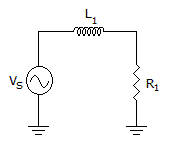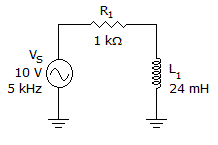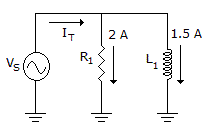# Electronics - RL Circuits

### Exercise :: RL Circuits - Filling the Blanks

1.If the resistance is larger than the inductive reactance in the given circuit, the phase angle is _______.

 A. less than 0° B. between 0° and 45° C. between 45° and 0° D. greater than 90°

Explanation:

No answer description available for this question. Let us discuss.

2.The phase angle in the circuit in the given circuit is approximately equal to ______.

 A. 53° B. 90° C. 37° D. 0°

Explanation:

No answer description available for this question. Let us discuss.

3.If VS increases in the given circuit, then the _____.

 A. impedance increases B. real power decreases C. phase angle increases D. current increases

Explanation:

No answer description available for this question. Let us discuss.

4.

The frequency at which XL = R in an RL circuit is called the _______________.

 A. cutoff frequency B. optimum frequency C. bandwidth characteristic D. resonant frequency

Explanation:

No answer description available for this question. Let us discuss.

5.In the given circuit, IT equals _____.

 A. 0.5 A B. 2 A C. 1.5 A D. 2.5 A